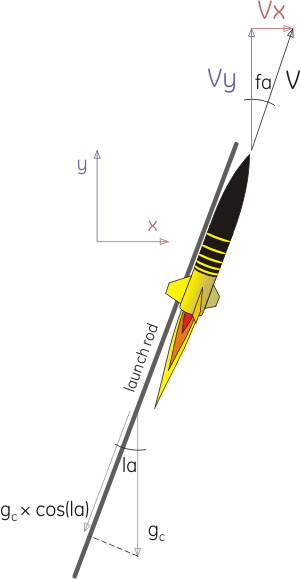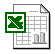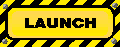Rocket Simulations 2D: Launch Angle

## Related Pages

• Rocket Simulations: the 1D (one-dimensional) simulation -- Note: you should be familiar with the 1D simulation before trying to tackle the 2D version.
• Rocket Equations: direct analytical solution for velocity and altitude - compute peak altitude without the need for simulation.Calculating altitude for a vertical launch is a one-dimensional problem, straight up and straight down. There is a single distance, a single velocity, a single thrust force and so on. When launching at an angle, we now have both a vertical and a horizontal component for each of the calculated parameters - distance, velocity, acceleration, thrust and drag. It is helpful to track the flight angle as well - the direction of the velocity vector. Notice I define two angles -- the launch angle, "la", the angle of the launch rod from vertical, which does not change (this is a constant) , and the flight angle, "fa", the angle of the rocket's flight path from vertical, which does change once the rocket is off the launch rod. The basic algorithm is the same as the vertical launch version of the simulation with the difference being that the horizontal and vertical components are found separately for each time increment and then combined to get a new velocity vector for that time point. If you are not familiar with vectors (values with both magnitude and direction) and vector arithmetic, you should study up on that topic so you can understand what's going on here. We'll skip the initial launch for the moment, and take up the algorithm mid-flight. Flight angle: the flight angle from the previous time increment was found from the velocity components, horizontal velocity Vx and vertical velocity Vy. The flight angle is fa = arctangent(Vx/Vy). The drag force Fd is found in the same way as the one dimensional method, using magnitude of the velocity vector V = sqrt(Vx^2+Vy^2). Then Fd = 0.5*rho*Cd*Area*V^2. This drag force is broken into horizontal (Fdx), and vertical (Fdy) components, as Fdx = Fd*sin(fa); Fdy = Fd*cos(fa). IMPORTANT NOTE: the value of fa that is used is the one from the previous time interval, not this time interval (otherwise the calculation becomes circular). The thrust Ft is given from the motor specs and handled in the same manner as with the 1D simulation, except once again we find a horizontal and vertical component: Ftx = Ft*sin(fa); Fty = Ft*cos(fa). Likewise using fa from previous interval. The total force F is found by first finding the horizontal component Fx and the vertical component Fy. Fx = Ftx - Fdx and Fy = Fty - Fdy - M*gc. The magnitude of the force vector can be found from F = sqrt(Fx^2 + Fy^2) -- this doesn't need to be calculated but can be for interest's sake. Acceleration is found separately in the horizontal and vertical directions. To find acceleration from force we need the rocket mass M, found for each time interval in the same manner as in the 1D method. Then horizontal acceleration is Accx = Fx/M and vertical acceleration is Accy = Fy/M. Velocity is then found from acceleration. The velocity from the previous interval is added to acceleration multiplied by the length of a time interval. So Vx = Vx(previous) + Accx*dt; Vy = Vy(previous) + Accy*dt. The magnitude of the velocity vector is V = sqrt(Vx^2 + Vy^2) and is needed, among other things, for the drag calculation in the next time interval. The new flight angle fa is then calculated from the velocity components Vx & Vy for this time interval as described in step 1. Note that the fins will track the direction of flight, so the thrust for the next time interval will now be directed along the new flight angle. Likewise the drag will be pointed on the same line in the opposite direction as the velocity. The x position (horizontal) is found as X = X(previous) + Vx*dt, and the vertical position found as Y = Y(previous) + Vy*dt. Note that, as with the 1D simulation, you can be a bit more precise by using X = X(previous) + Vx*dt + 0.5*Accx*dt^2 and similarly for vertical Y = Y(previous) + Vy*dt + 0.5*Accy*dt^2, which is more correct and most often will not make much difference. There's one other trick -- handling the effect of the launch rod during the initial launch. The launch rod only allows forces, like gravity, to act along the axis of the rod. Since the rocket is aligned with the rod, the full thrust is seen along that axis. The component of gravity that is perpendicular to the rod is supported by the rod, so only the component along the axis of rod acts on the rocket during this phase. This would be gc*cos(la), where la is the launch angle. Because this is no longer vertical, there is a horizontal and vertical component, so the horizontal component is gx = gc*cos(la)*sin(la) and the vertical component is gy = gc*cos(la)*cos(la) = gc*cos(la)^2. Then until the rocket reaches the end of the launch rod, Fx = Ftx - Fdx - M*gx Fy = Fty - Fdy - M*gy I handle this in the spreadsheet by defining gx & gy as above until the rocket's travel exceeds the length of the launch rod, then past that point, gx = 0 and gy = gc.

## Need Some Examples?My MiniMagg Excel Simulation, high power 2D sim using thrust curves, with trial & error launch angle optimizing for wind speed.Mark Sullivan's Model Rocket Altitude Predictor: an outstanding, working online rocket simulator

## Questions

Your questions and comments regarding this page are welcome. You can e-mail Randy Culp for inquiries, suggestions, new ideas or just to chat.
Updated 19 July 2009